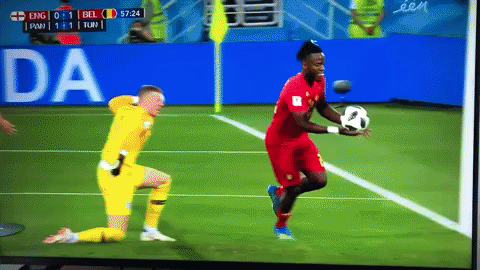After posting a couple of my World Cup viz on Twitter, I thought I’ll collate some of them into a blog post. This will be Part 1 of a series as the World Cup goes on and I keep improving my viz skills throughout the tournament. I will also explain how I made improvements in each new plot, practice makes perfect!

Let’s look at some of the packages I will use!

library(ggplot2)   # plotting on top of ggsoccer
library(ggsoccer)  # create soccer pitch overlay
library(dplyr)     # data manipulation
library(purrr)     # create multiple dataframes for tweenr
library(tweenr)    # build frames for animation
library(gganimate) # animate plots
library(extrafont) # insert custom fonts into plots
library(ggimage)   # insert images and emoji into plots


The important package here is the ggsoccer package made by Ben Torvaney, check out the GitHub repo here.

Showing is better than telling in this instance so let’s take a look at the pitch:

library(ggplot2)
library(ggsoccer)

data <- data.frame(x = 1, y = 1)

ggplot(data) +
annotate_pitch() +
theme_pitch() +
coord_flip(xlim = c(49, 101),
ylim = c(-1, 101))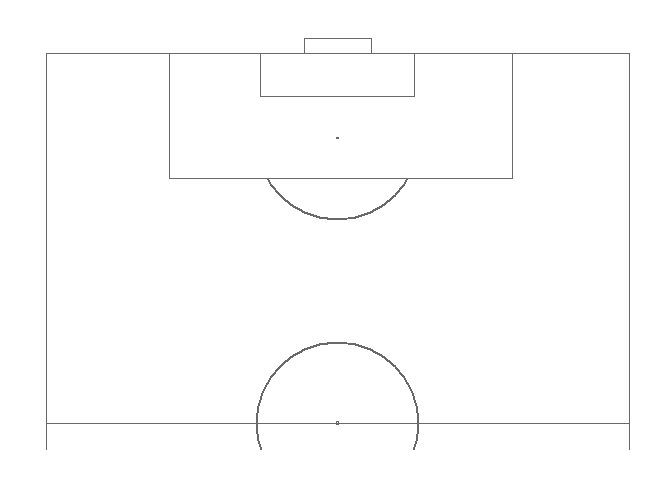Basically, annotate_pitch() creates the markings for the soccer field such as the center circle, 18-yard box, penalty spot, etc. while theme_pitch() erases the extraneous axes and background from the default ggplot style. By using the limits arguments in coord_flip(), we can focus on a certain area of the pitch and orient it in a way that we want, as I want to recreate goals I’m going to show only one half of the field and orient the view to face the goal. With this as the base, we can now input positional data and then use a combination of geom_segment() and geom_curve() to show the path of the ball and the players!

The only problem with doing this is manually creating the data points. This is more a problem of access to the data rather than availability as sports analytics firms, most notably Opta, generate a huge amount of data for every player in every match, however it is not easy for a regular guy like me to buy it.

Some people have managed to create some nice heatmaps by scraping WhoScored.com and other sites (that create their viz from purchased data from Opta) with RSelenium or some other JS scrapers but that was a bit out of my expertise so I resorted to creating the coordinate positions by hand. Thankfully, due to the plotting system in ggsoccer and ggplot2, it’s very easy to figure out the positions on the soccer field plot and with a little bit of practice it doesn’t take too much time.To save space I don’t show the data frames with the coordinate points and labelling data for all of the graphics, however you can find all of them here in the GitHub repo!

### Gazinsky Scores The First Goal!

ggplot(pass_data) +
annotate_pitch() +
theme_pitch() +
theme(text = element_text(family = "Trebuchet MS")) +
coord_flip(xlim = c(49, 101),
ylim = c(-1, 101)) +
geom_segment(aes(x = x, y = y, xend = x2, yend = y2),
arrow = arrow(length = unit(0.25, "cm"),
type = "closed")) +
geom_segment(data = ball_data,
aes(x = x, y = y, xend = x2, yend = y2),
linetype = "dashed", size = 0.85,
color = c("black", "red")) +
geom_segment(data = movement_data,
aes(x = x, y = y, xend = x2, yend = y2),
linetype = "dashed", size = 1.2,
color = "darkgreen") +
geom_curve(data = curve_data,
aes(x = x, y = y, xend = x2, yend = y2),
curvature = 0.25,
arrow = arrow(length = unit(0.25, "cm"),
type = "closed")) +
geom_image(data = goal_img,
aes(x = x, y = y,
image = image),
size = 0.035) +
ggtitle(label = "Russia (5) vs. (0) Saudi Arabia",
subtitle = "First goal, Yuri Gazinsky (12th Minute)") +
labs(caption = "By Ryo Nakagawara (@R_by_Ryo)") +
geom_label(data = label_data,
aes(x = x, y = y,
label = label,
hjust = hjust,
vjust = vjust)) +
annotate("text", x = 69, y = 65, family = "Trebuchet MS",
label = "After a poor corner kick clearance\n from Saudi Arabia, Golovin picks up the loose ball, \n exchanges a give-and-go pass with Zhirkov\n before finding Gazinsky with a beautiful cross!")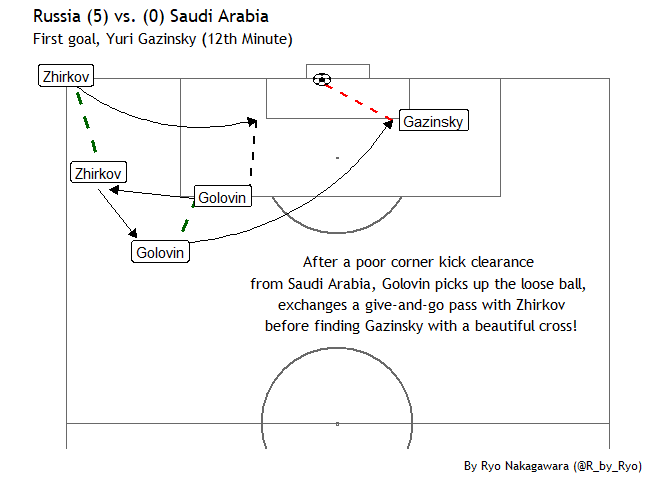Not bad for a first try. Let’s take a closer look at how I plotted the soccer ball image into the plot.

goal_img <- data.frame(x = 100,
y = 47) %>%
mutate(image = "https://d30y9cdsu7xlg0.cloudfront.net/png/43563-200.png")

## ggplot2 code ##
geom_image(data = goal_img,
aes(x = x, y = y,
image = image),
size = 0.035)
## ggplot2 code ##


I used the ggimage package to be able to create a geom layer for an image. I created a column called image in a dataframe with the URL link to the soccer ball image I wanted and then in the geom_image() function I specified it in the image argument.

## Cristiano’s Hattrick!

In my excitement after seeing Portugal vs. Spain, a candidate for match of the tournament for the group stages if not for the whole tournament, I drew up Cristiano Ronaldo’s hattrick!

ggplot(goals_data) +
annotate_pitch() +
theme_pitch() +
theme(text = element_text(family = "Dusha V5"),
legend.position = "none") +
coord_flip(xlim = c(55, 112),
ylim = c(-1, 101)) +
geom_segment(x = 80, y = 48,
xend = 97, yend = 48) +  # 2nd
geom_segment(x = 97, y = 48,
xend = 100, yend = 45.5,
arrow = arrow(length = unit(0.25, "cm"),
type = "closed")) +        # degea fumble
geom_curve(data = curve_data,
aes(x = x, y = y,
xend = xend, yend = yend),     # FREEKICK
curvature = 0.3,
arrow = arrow(length = unit(0.25, "cm"), type = "closed")) +
geom_text(data = annotation_data,
family = "Dusha V5",
aes(x = x, y = y,
hjust = hjust, label = label),
size = c(6.5, 4.5, 3, 3.5, 3.5, 3.5)) +
geom_flag(data = flag_data,
aes(x = x, y = y,
image = image), size = c(0.08, 0.08)) +       # Portugal + Spain Flag
ggimage::geom_emoji(aes(x = 105,
y = c(45, 50, 55)),
image = "26bd", size = 0.035) +
geom_point(aes(x = x, y = y),
shape = 21, size = 7, color = "black", fill = "white") +
geom_text(aes(x = x, y = y, label = label, family = "Dusha V5"))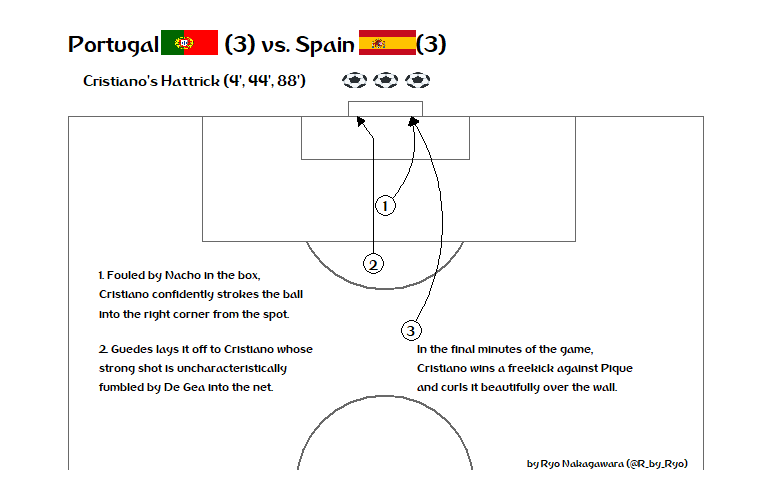Compared to the first plot, I increased the x-axis limit so that we could place our geom_text() annotations and flag images together without having to use grobs. This also meant that we put the plot title and subtitle in the geom_text() rather than in the labs() function, which let all the text/label data be organized in one dataframe, annotation_data.

annotation_data <- data.frame(
hjust = c(0.5, 0.5, 0.5, 0, 0, 0),
label = c("Portugal             (3) vs. Spain            (3)",
"Cristiano's Hattrick (4', 44', 88')",
"by Ryo Nakagawara (@R_by_Ryo)",
"1. Fouled by Nacho in the box,\nCristiano confidently strokes the ball\ninto the right corner from the spot.",
"2. Guedes lays it off to Cristiano whose\nstrong shot is uncharacteristically\nfumbled by De Gea into the net.",
"In the final minutes of the game,\nCristiano wins a freekick against Pique\nand curls it beautifully over the wall."),
x = c(110, 105, 53, 76, 66, 66),
y = c(30, 20, 85, 5, 5, 55)
)


Overall, it’s a slightly hacky solution to include a lot of blank spaces between the country name and the score to put the flags in between them, but I don’t know of any geoms that can incorporate both text and images at the same time so the hacky solution will do!

To show the flags I use the geom_flag() function from the ggimage package. The function requires you to pass a two-digit ISO code in the image argument for the flags of the countries you want. You can find the ISO codes for countries with a quick google search, Portugal is “PT” and Spain is “ES”.

flag_data <- data.frame(
image = c("PT", "ES"),
x = c(110, 110),
y = c(19.1, 50.1)
)

## ggplot2 code ##
geom_flag(data = flag_data,
aes(x = x, y = y,
image = image, size = size))
## ggplot2 code ##


Some other options to do this include using the ggflags package or if you don’t like the flags used in geom_flag(), pass an image of a flag of your choosing to geom_image().

There is actually a better way to search for the ISO codes which I will show later!

This time, instead of the soccer ball image, I used the emoji_search() function from the emoGG package to find a soccer ball emoji. Then I can use either emoGG or ggimage’s geom_emoji() function to insert it into my ggplot!

library(emoGG)
library(ggimage)

emoji_search("soccer") # "26bd"

##        emoji             code  keyword
## 2537  soccer             26bd   sports
## 2538  soccer             26bd football
## 4130       o             2b55   circle
## 4131       o             2b55    round
## 5234 eritrea 1f1ea\\U0001f1f7       er
## 5956 somalia 1f1f8\\U0001f1f4       so

## ggplot2 code ##
ggimage::geom_emoji(aes(x = 105,
y = c(45, 50, 55)),
image = "26bd", size = 0.035)
## ggplot2 code ##


From now on, instead of the soccer ball image in the first graphic, I will be using the emoji version!

The official World Cup font, “Dusha”, was created by a Portugese design agency back in 2014 and has been used in all official World Cup prints and graphics. Some of the letters may look a bit squished but overall I quite like it, so I wanted to incorporate it in my plots. To do so you need to download the .TTF file from here, then right-click and install it. Now, we need to make sure R can use it, this can be done by using the extrafont package!

font_import()  # import font files in your computer

loadfonts()    # run every new session once!

fonts()        # to check out what fonts are ready for use in R!


For more details check out the package README here. Again, remember to run loadfont() everytime you open up a new session!

## Osako’s Winner vs. Colombia

I wasn’t expecting much from Japan’s World Cup journey this time around due to our poor performances in the friendlies (besides the Paraguay game) and the fact that we changed our manager in April! However, with a historic win (our first against South American opposition in the World Cup), I couldn’t resist making another R graphic:

library(ggplot2)
library(dplyr)
library(ggsoccer)
library(extrafont)
library(ggimage)
library(countrycode)

cornerkick_data <- data.frame(x = 99, y = 0.3,
x2 = 94, y2 = 47)

osako_gol <- data.frame(x = 94, y = 49,
x2 = 100, y2 = 55.5)

player_label <- data.frame(x = c(92, 99),
y = c(49, 2))

annotation_data <- data.frame(
x = c(110, 105, 70, 92, 53),
y = c(30, 30, 45, 81, 85),
hjust = c(0.5, 0.5, 0.5, 0.5, 0.5),
label = c("Japan             (2) vs. Colombia             (1)",
"Kagawa (PEN 6'), Quintero (39'), Osako (73')",
"Japan press their man advantage, substitute Honda\ndelivers a delicious corner kick for Osako to (somehow) tower over\nColombia's defense and flick a header into the far corner!",
"Bonus: Ospina looking confused and\ndoing a lil' two-step-or-god-knows-what.",
"by Ryo Nakagawara (@R_by_Ryo)")
)

flag_data <- data.frame(
x = c(110, 110),
y = c(13, 53),
team = c("japan", "colombia")
) %>%
mutate(
image = team %>%
countrycode(., origin = "country.name", destination = "iso2c")
) %>%
select(-team)

wc_logo <- data.frame(x = 107,
y = 85) %>%

ggplot(osako_gol) +
annotate_pitch() +
theme_pitch() +
theme(text = element_text(family = "Dusha V5"),
plot.margin=grid::unit(c(0,0,0,0), "mm")) +
coord_flip(xlim = c(55, 112),
ylim = c(-1, 101)) +
geom_curve(data = cornerkick_data,
aes(x = x, y = y, xend = x2, yend = y2),
curvature = -0.15,
arrow = arrow(length = unit(0.25, "cm"),
type = "closed")) +
geom_segment(aes(x = x, y = y, xend = x2, yend = y2),
arrow = arrow(length = unit(0.25, "cm"),
type = "closed")) +
geom_label(data = player_label,
aes(x = x, y = y),
label = c("Osako", "Honda"), family = "Dusha V5") +
geom_point(aes(x = 98, y = 50), size = 3, color = "green") +
geom_text(aes(x = 99.7, y = 50), size = 5, label = "???", family = "Dusha V5") +
geom_text(data = annotation_data,
family = "Dusha V5",
aes(x = x, y = y,
hjust = hjust, label = label),
size = c(6.5, 4.5, 4, 3.5, 3)) +
ggimage::geom_flag(data = flag_data,
aes(x = x, y = y,
image = image),
size = c(0.08, 0.08)) +
ggimage::geom_emoji(aes(x = 95,
y = 50),
image = "26bd", size = 0.035) +
geom_image(data = wc_logo,
aes(x = x, y = y,
image = image), size = 0.17)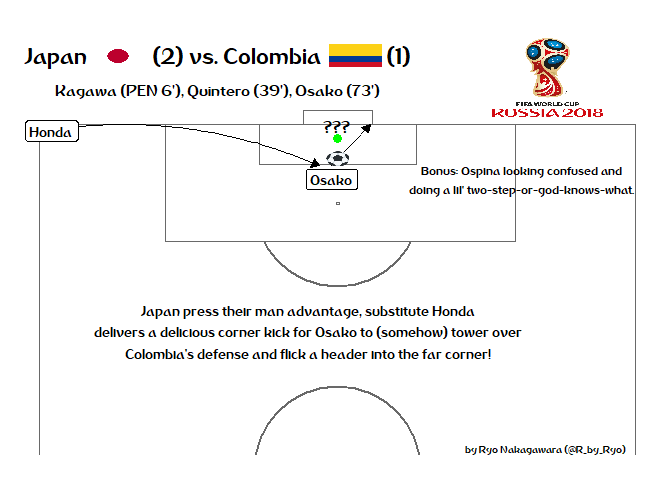I could have used the annotate() function to add the little comment about Ospina being stuck in no-man’s-land but I prefer to have all of my text in a single dataframe. Like before, I again had to expand the x-axis limits in the coord_flip(). This is also so we can insert the World Cup image on the top right without using grobs/Magick and such. To grab that World Cup logo, we do the same things as we did when we added the soccer ball image in the first plot with ggimage.

For finding the ISO codes to input for the geom_flag() function we can do one better than previous attempts by using the countrycode package to find ISO codes without having to manually search online!

By passing country names into countrycode() function and labelling them as “country.name” in the origin argument, the function will know that the input is the regular name for a country. Then you specify the output such as “iso2c” for the two-digit ISO codes such as in our case, “wb” for World Bank codes, “eurostat.name” for country names in the Eurostat database and so on…!

library(countrycode)

flag_data <- data.frame(
x = c(110, 110),
y = c(13, 53),
team = c("japan", "colombia")
) %>%
mutate(
image = team %>%
countrycode(., origin = "country.name", destination = "iso2c")
) %>%
select(-team)

glimpse(flag_data)

## Observations: 2
## Variables: 3
## $x <dbl> 110, 110 ##$ y     <dbl> 13, 53
## \$ image <chr> "JP", "CO"


Although the ISO codes are pretty intuitive for countries like Japan and Colombia, when you’re dealing with lots of countries like at the World Cup or the Olympics, having a reproducible workflow for this is very helpful!

In a future part (not necessarily the next part), I want to animate some of these goal graphics using the great gganimate and tweenr packages. I’ve been slowly working my way through them in the past week so here is a preview:

I’ll only show a gganimate version of Gazinsky’s goal for now as I’m still figuring out how to interpolate multiple moving objects (the ball and the players) as well as making the green movement lines disappear after the player finished moving.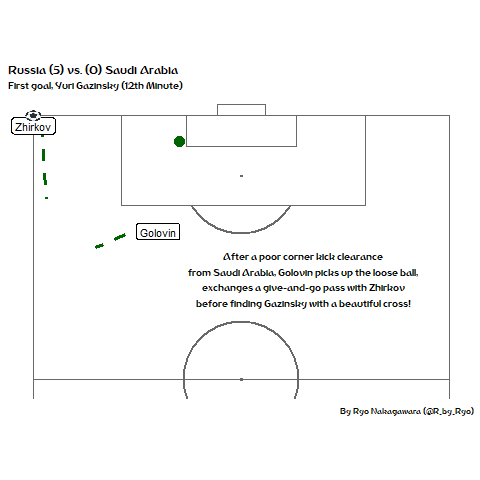For Osako’s goal, here’s a preview of the tweenr version. Working on this was much easier as I had made it so that the only moving bit to interpolate was the path of the ball.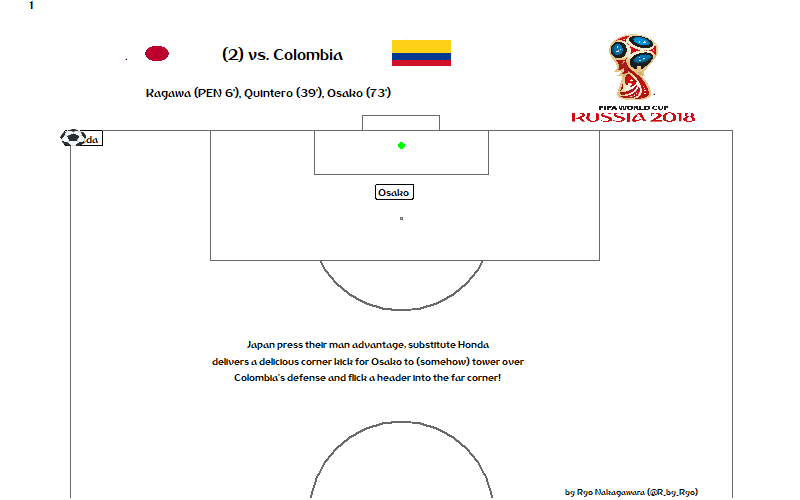I’ve been playing around a lot with the different easing functions using this website as a reference but it still doesn’t feel 100% right… For this one I used “quadratic-out”. I want to make sure that the ball doesn’t completely come to a full stop when it reaches Osako but most keep doing that.

These goals are just from the first week of the World Cup and if I had the time I would do more as there have been some fantastic individual and team goals so far!

With the Group Stages done, I am looking forward to an even more exciting Knockout Stage, good luck to all of your favorite teams!

And hopefully no own goals: